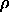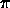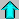# Astronomy 12 - Spring 1999 (S.T. Myers)

## Problem Set #2 (due Thu 4 Feb 1999 5pm)

1. We have been discussing binary stars, but a large planetary companion to a Sun-like star could be found using the same methods we have discussed for binary stars. The discovery of extrasolar planets and brown dwarf companions in an important growing area in astronomy, and the following two problems will illustrate the difficulties.

In Problem Set 1, we modeled Jupiter as a blackbody with a temperature of 122 K. Assuming that the radius of Jupiter is 71400 km, calculate the bolometric luminosity of Jupiter. Compare this to the luminosity of the Sun.

At what wavelength will the maximum of the blackbody spectrum be (in microns) for the Sun and for Jupiter?

If we wanted to discover a Jupiter-like planet near a distant Sun-like star, this is how much fainter it would appear! If the absolute bolometric magnitude of the Sun is +4.75, then what is the absolute bolometric magnitude of Jupiter?

The Hubble Space Telescope (HST) can detect objects with apparent magnitudes of m = +30 or less (brighter), though it may take exposures of up to hundreds of orbits like the Hubble Deep Field. Would Jupiter be bright enough to see with HST from 10 pc away? (For the purposes of this question, we will ignore the fact that the peak of the luminosity appears in the infrared while HST is most sensitive in the visible band.)

The angular resolution of the HST is 0.1'', and thus HST would be able to separate the images if they were separated by 0.1'' or more. What is the maximum distance in parsecs at which HST could resolve the Sun and Jupiter at their relative separation of 5.2 AU?

2. Rather than detecting the Sun and Jupiter visually, one could hope to detect the orbital velocity of the star produced by the reflex motion with respect to the planet's orbit. Calculate the orbital velocity (m/s) of Jupiter in its 11.86 year orbit with semimajor axis of 5.203 AU from the Sun (assume the orbit is approximately circular). If the mass of Jupiter is 1.9 x 10^27 kg, what is the corresponding orbital velocity (m/s) of the Sun around the Sun-Jupiter barycenter (ignore the presence of all the other planets)?

If current spectroscopic technology limits the measurement of radial velocities to 1 m/s or greater, then at what maximum orbital distance (in AU) could the reflex motion of the Sun from a Jupiter-mass planet be detected with a velocity of 1 m/s or more? What about an Earth-mass planet around a Solar-mass star?

3. The star Zeta Phoenicis is a 1.67 day spectroscopic binary with nearly circular orbits. The maximum measured Doppler shifts of the primary and secondary stars are 121.4 km/s and 247 km/s respectively. Calculate the total mass function

f(M) = M sin3i

and the individual masses m_1 sin^3i and m_2 sin^3i for the components of this system.

4. Recent observations uncovered a double-line eclipsing spectroscopic binary star system in the little-known constellation Linus Segmentus. The pair have a period of 8 years and the total orbital radial velocity is 29.86 km/s, the same as the orbital velocity of the Earth around the Sun. The radial velocity of the primary star Itchy is 1/15 of the radial velocity of the secondary star Scratchy. The eclipses have flat minima and are well separated. Find the total mass of the system in solar masses M_sun as well as the individual masses of Itchy and Scratchy.

Itchy and Scratchy are also a visual binary, with a maximum separation on the sky of 0.2''. What is the distance, in parsecs, to the system?

The apparent bolometric magnitude M_bol of the fainter star Scratchy is observed to be +10.4, while that of the brighter Itchy is -0.5 magnitudes. The spectral temperature of Itchy is 18700 K, and that of Scratchy is 3850 K. Find the absolute bolometric magnitudes of Itchy and Scratchy, their luminosities (in L_sun), and their radii (in R_sun).

5. We return to examining the binary system Sirius A and B. From the previous problem set, you should have found the masses (in solar masses M_sun) and luminosities (in solar luminosities L_sun) of Sirius A and B. You should note those down here, or go back and recalculate them. At the end of the problem, we noted that the M/L for these stars were very different.

Observations of the spectra of the two stars show that Sirius A has an effective blackbody temperature of 9200 K, and Sirius B has a temperature of 27000 K (as compared the Sun's temperature of 5770 K). Use the relationship between surface flux, luminosity and temperature to calculate the radii of Sirius A and B. Compare these to the Sun's radius. Also comment on the inferred small radius of Sirius B (you might try comparing it to the size of the Earth).

Use the masses and radii to calculate the mean densities of Sirius A and B and the Sun (in kg/m^3). Remember for a sphere, the mean density is given by

 3 M= __________ 4R3

Compare these to the mean density of the planet Earth for example. Comment on the density of Sirius B.Astr12 Index ---Astr12 Home

smyers@nrao.edu Steven T. Myers